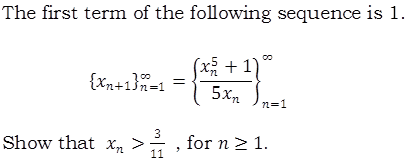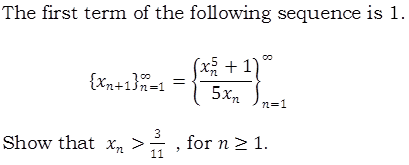# Lower Bound of Sequence

## Homework Statement## The Attempt at a Solution

This is what I have so far:
$$x_{n+1}=\frac{x^5_n + 1}{5x_n}=1$$

$$x_{n+2}=\frac{x^5_{n+1} + 1}{5x_{n+1}}=\frac{1^5+1}{5}$$

I think you have to do some sort of repeated substitution but I don't quite see it. Any help? Thanks.

HallsofIvy
Homework Helper

## Homework Statement## The Attempt at a Solution

This is what I have so far:
$$x_{n+1}=\frac{x^5_n + 1}{5x_n}=1$$
Why would this be equal to 1? As far as you know to start with, only x1 is equal to 1 and then
$x_2= 2/5$, $x_3= (32/3125+ 1)/2= 1580/3125= 316/625'$ which is about 0.5056. x1 is the only term that is equal to 1.

$$x_{n+2}=\frac{x^5_{n+1} + 1}{5x_{n+1}}=\frac{1^5+1}{5}$$

I think you have to do some sort of repeated substitution but I don't quite see it. Any help? Thanks.
Use induction. You already know that $x_1= 1> 3/11$. Show that if $x_n> 3/11$ then $x_{n+1}= (x_n^5+ 1)/5x_n> 3/11$.

I meant to say $$x_{1+1}=\frac{x^5_1 + 1}{5x_1}=1$$
Since the first term of the sequence on the left is $$x_{((1)+1)}$$

won't it be $x_2=1$ and not $x_1=1$?

Thanks.

haruspex
Homework Helper
Gold Member
2020 Award
Since the first term of the sequence on the left is $$x_{((1)+1)}$$
No, the sequence is defined from n=1, so the first term is x1. You are confusing that with the first opportunity to use the recurrence relation.

No, the sequence is defined from n=1, so the first term is x1. You are confusing that with the first opportunity to use the recurrence relation.

The notation is confusing me. The problem is equating two different sequences, right? And the sequence on the left is defined as xn+1 from n=1.

So for n=1: xn+1 = x(1)+1 = x2

Isn't this the first term?

Last edited:
Never mind; silly me. It is the definition of a recursive sequence.

I don't understand how exactly to prove by induction. Can I do the following?

$$x_{n+1}> ((3/11)^5+ 1)/5(3/11)= 0.74> 3/11$$

haruspex
Homework Helper
Gold Member
2020 Award
Never mind; silly me. It is the definition of a recursive sequence.

I don't understand how exactly to prove by induction. Can I do the following?

$$x_{n+1}> ((3/11)^5+ 1)/5(3/11)= 0.74> 3/11$$
No, that's not valid. What would happen to the bound on xn+1 if you made xn a bit larger? The numerator is ok, that would just boost xn+1 a bit more, but the denominator goes up too, and it's not obvious what the net effect is.
You need to show that x > 3/11 implies (x5+ 1)/5x > ((3/11)5+ 1)/5(3/11)

Unfortunately, I don't understand why that is implied.

Is this a valid solution?

$$x_n = \frac{x_{n-1}^5 +1}{5x_{n-1}}\\ f(x) = \frac{x^5+1}{5x}$$

Find the minimum using the first derivative.

$$f '(x)=\frac{4x^5-1}{5x^2}\\ f '(x)=0\\ x=(\frac{1}{4})^\frac{1}{5}\\ f(x) = \frac{((\frac{1}{4})^\frac{1}{5})^5+1}{5((\frac{1}{4})^\frac{1}{5})} \approx 0.33 \\ \frac{3}{11} \approx 0.273$$

The lowest possible value is greater than 3/11.

haruspex